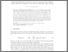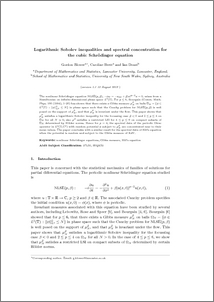# Logarithmic Sobolev inequalities and spectral concentration for the cubic Shrödinger equation

Blower, Gordon and Brett, Caroline and Doust, Ian (2014) Logarithmic Sobolev inequalities and spectral concentration for the cubic Shrödinger equation. Stochastics, 86 (6). pp. 870-881. ISSN 1744-2508Preview
PDF (logsobnlseLatb)
logsobnlseLatb.pdf - Submitted Version

## Abstract

The nonlinear Schr\"odinger equation $\NLSE(p, \beta)$, $-iu_t=-u_{xx}+\beta \vert u\vert^{p-2} u=0$, arises from a Hamiltonian on infinite-dimensional phase space $\Lp^2(\mT)$. For $p\leq 6$, Bourgain (Comm. Math. Phys. 166 (1994), 1--26) has shown that there exists a Gibbs measure $\mu^{\beta}_N$ on balls $\Omega_N= \{ \phi\in \Lp^2(\mT) \,:\, \Vert \phi \Vert^2_{\Lp^2} \leq N\}$ in phase space such that the Cauchy problem for $\NLSE(p,\beta)$ is well posed on the support of $\mu^{\beta}_N$, and that $\mu^{\beta}_N$ is invariant under the flow. This paper shows that $\mu^{\beta}_N$ satisfies a logarithmic Sobolev inequality for the focussing case $\beta <0$ and $2\leq p\leq 4$ on $\Omega_N$ for all $N>0$; also $\mu^{\beta}$ satisfies a restricted LSI for $4\leq p\leq 6$ on compact subsets of $\Omega_N$ determined by H\"older norms. Hence for $p=4$, the spectral data of the periodic Dirac operator in $\Lp^2(\mT; \mC^2)$ with random potential $\phi$ subject to $\mu^{\beta}_N$ are concentrated near to their mean values. The paper concludes with a similar result for the spectral data of Hill's equation when the potential is random and subject to the Gibbs measure of KdV.

Item Type:
Journal Article
Journal or Publication Title:
Stochastics
Submitted for publication, and posted on ArXiv 1308:3649 The final, definitive version of this article has been published in the Journal, Stochastics, 86 (6), 2014, © Informa Plc
Uncontrolled Keywords:
/dk/atira/pure/subjectarea/asjc/2600
Subjects:
Departments:
ID Code:
66185
Deposited By:
Deposited On:
27 Aug 2013 12:09
Refereed?:
Yes
Published?:
Published